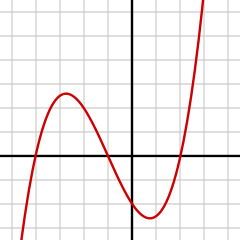Calculus

# Differentiability: Level 3 Challenges$\large{x^3+x^2-5x-1=0}$

Let $\alpha,\beta,\gamma$ be the roots of the equation above.

Evaluate $\left\lfloor \alpha \right\rfloor +\left\lfloor \beta \right\rfloor +\left\lfloor \gamma \right\rfloor$.

###### Image Credit: Wikimedia N.Mori.

Let $f$ be a twice differentiable function satisfying $f(1)=1, f(2)=4, f(3)=9$, then :

Let $0. If $f\left( x \right) =\left| \begin{matrix} \sin { x } & \sin { a } & \sin { b } \\ \cos { x } & \cos { a } & \cos { b } \\ \tan { x } & \tan { a } & \tan { b } \end{matrix} \right|$ , then minimum possible number of roots of $f'\left( x \right) =0$ lying in $(a,b)$ is:

Find the equation of common tangent to the curves $y^2=8x$ and $xy=-1$?

The curve of $\sin { \left( ax \right) }$ is tangent to the curve of $\sin { \left( x \right) }$ at $x=\frac { 5\pi }{2 }$.

If minimum positive value of $a$ can be expressed as $\frac { A }{B }$ for co-prime $A$ and $B$, then find $A+B$.

×